# 1 Full information extracted from a Covariance matrix

This brief summary is mainly thought for my students of Multivariate Statistic. I am writing some topics directly in english.

## 1.1 Information contained in the Covariance Matrix.

The Covariance Matrix (also named Variance Matrix, or Variance-Covariance Matrix) is the second order multivariate centered moment of a multiple random variable .

Many of the results here recalled can be extended to covariance matrices of multivariate observations.

### 1.1.1 Information obtained from the first and second multivariate moments

The first and second multivariate moments, that is the vector of the mathematical expectations and the covariance matrix, they contain all (and only) the information needed to analyze all types of linear correlations related to pairs or groups of variables, both in marginal and conditional distributions.

I summarize the linear relationships in connection with the first and second moments, according to what has been studied so far, for multiple random variables $$\mathbf{Y}$$ with $$p$$ components and with first moment equal to $$\boldsymbol{ \mu}$$, so that we have:

$\mathbf{Y}=\left\{X_1,X_2,\dots,X_{i},\dots,X_{p}\right\}^{\mathsf{T}} \qquad \mbox{with} \qquad$ $\mathrm{E}\left[\mathbf{Y}\right]=\boldsymbol{ \mu}\qquad \mbox{and} \qquad \mathrm{V}\left[\mathbf{Y}\right]=\mathrm{E}\left[(\mathbf{Y}-\boldsymbol{ \mu}) (\mathbf{Y}-\boldsymbol{ \mu})^{\mathsf{T}}\right]=\boldsymbol{ \Sigma}$ where $\mu_j=\mathrm{E}\left[Y_j\right] \qquad \sigma_{ij}=\left\{\boldsymbol{ \Sigma}\right\}_{ij}=\mathrm{E}\left[(Y_i-\mu_i)(Y_j-\mu_j)\right]$

I denote with $$\boldsymbol{ \Sigma}$$ the variance covariance matrix and with $$\mathbf{R}$$ the correlation matrix, defined as usual:

$r_{ij} =\frac{\sigma_{ij}}{\sigma_{i}\sigma_{j}}$

where $$\sigma_{i}^{2}$$ is the variance of the $$i$$-th components, that is the $$i$$-th -diagonal element of $$\boldsymbol{ \Sigma}$$ (and $$\sigma_{i}$$ is the standard deviation of $$i$$-th variable)

If $$D$$ is a diagonal matrix of generic element $$d_{ij}$$ such that: $d_{ij}= \left\{ \begin{array}{ccc} 0 & \mbox{if} & i \neq j \\ \sigma_{i}^{2} & \mbox{if} & i = j\\ \end{array} \right.$ then the correlation matrix $$\mathbf{R}$$ is given by:

$\mathbf{R}= D^{-\frac{1}{2}} \boldsymbol{ \Sigma}D^{-\frac{1}{2}},$ (of course $$\mathbf{R}$$ is the covariance matrix of standardized variables)

Supposing that the inverse of $$\boldsymbol{ \Sigma}$$ exists, that is: $$\left|\boldsymbol{ \Sigma}\right| \ne 0$$, let’s denote such inverse with $$\mathbf{C}$$, of generic element $$c_{ij}$$, so that:

$\mathbf{C}=\boldsymbol{ \Sigma}^{-1} \qquad \mbox{and} \qquad c_{ij}=\frac{\sigma^{ij}}{\left|\boldsymbol{ \Sigma}\right|}$ where $$\sigma^{ij}$$ is the cofactor of place $$i,j$$ of $$\boldsymbol{ \Sigma}$$

### 1.1.2 Meaning of the elements of Covariance matrix and Correlation matrix

$$\sigma_{i}^{2}$$: the variance of a component $$Y_{i}, \quad i=1,2,\dots,p$$

Covariance matrix of a linear combination $$\mathbf{Z}= \mathbf{A}\mathbf{Y}$$

$\mathrm{V}\left[\mathbf{Z}\right]=\mathbf{A} \boldsymbol{ \Sigma} \mathbf{A}^{\mathsf{T}}$

The trace of the matrix $$\boldsymbol{ \Sigma}$$ is the total variance:

$$tr(\boldsymbol{ \Sigma})=\sum_{i=1}^{p}\sigma_{i}^{2}$$ total variance

$$\left|\boldsymbol{ \Sigma}\right|$$: generalized variance (Wilks variance) This multivariate measure of variability is proportional to the volume of the ellipsoid defined by the equation $$\mathbf{t},\boldsymbol{ \Sigma}^{\mathsf{T}} = \mathbf{t},\boldsymbol{ \Sigma}k$$. It is zero only if: $$rank(\boldsymbol{ \Sigma})<p$$, that is when there is an exact collinearity among the components of $$\mathbf{Y}$$. It can be zero even if every variance is positive… For a correlation matrix (that is, for standardized variables), this measure is maximized by independent components.

$$r_{ij} =\frac{\sigma_{ij}}{\sigma_{i}\sigma_{j}}$$: linear correlation between two components $$Y_{i}, Y_{j}$$

$$b_{i,j}=\frac{\sigma_{ij}}{\sigma_{j}^{2}}$$ linear regression coefficient the component $$Y_{i}$$ conditioned on $$Y_{j}$$.

### 1.1.3 Meaning of the elements of the inverse of the Covariance matrix and of the Correlation matrix

I recall main results (with $$\mathbf{C}=\boldsymbol{ \Sigma}^{-1}$$, also called precision matrix)

#### 1.1.3.1 Diagonal elements:

$$c_{ii}=\frac{1}{\sigma^2_i (1-R^2_{i.{B}})}$$ where $$R^2_{i.{B}}$$ is the multiple $$R^2$$ coefficient of the multiple linear regression of $$Y_i$$ depending on the remaining $$p-1$$ components.

($$B$$ is the set of indeces associated to remaining variables: $$B={1,2, \dots , j-1, j+1, \dots ,p}$$)

so that we have also: $R^2_{i.{B}}=1-\frac{1}{\sigma_{i}^{2} c{ii}}]$ $$R^2_{i.{B}}$$ is the maximum squared linear correlation between $$Y_i$$ and a linear combination of the remaining p-1 components $$\mathbf{Y}_B$$ (the best linear combination of $$\mathbf{Y}_B$$ according to least squares, with coefficients $$\boldsymbol{ \beta}$$)

#### 1.1.3.2 Diagonal elements of the inverse of a correlation matrix:

For standardized variables: $$1-\frac{1}{c_{ii}}$$ is the multiple $$R^2$$ coefficient of a variable from the remaining and diagonal elements $$c_{ii}$$ gives the inverse of residual variance of each component as a linear function of the remaining ones.

#### 1.1.3.3 Off-diagonal elements of the inverse

$r_{ij.B}=\frac{-c_{ij}}{\sqrt{c_{ii} c_{jj}}}$

partial correlation between two variables $$Y_{i}$$ and $$Y_{j}$$ keeping fixed the remaining $$p-2$$ (where now B is the set of all indeces excluding {i,j})

## 1.2 Eigenvalues and eigenvectors of the covariance matrix

Given the sequence of decreasing eigenvalues of $$\boldsymbol{ \Sigma}$$, $$\lambda_1\ge\lambda_2\ge\dots\ge\lambda_p$$:

$$\lambda_{1}$$ is the maximum variance of a linear combination of $$\mathbf{Y}$$ with normalized coefficients (the first eigenvector $$\boldsymbol{ \gamma} _1$$)

$$\lambda_{p}$$ is the minimum variance of a linear combination of $$\mathbf{Y}$$ with normalized coefficients (the last eigenvector $$\boldsymbol{ \gamma} _p$$) and ortogonal to the other sets of coefficients

### 1.2.1 An example

eigen() decomposition
$values  1.8390953 0.9376098 0.7349175 0.4883773$vectors
[,1]       [,2]       [,3]        [,4]
[1,] 0.5616631  0.4075851  0.2101585  0.68865258
[2,] 0.5857588  0.2067333  0.3428132 -0.70471769
[3,] 0.4871432 -0.1363376 -0.8609296 -0.05388706
[4,] 0.3226650 -0.8789470  0.3116291  0.16194687

### 1.2.2 Correspondence with an ellipse rotation

#### 1.2.2.1 Canonical correlations

only mentioned, can be obtained again from the covariance matrix and measures the maximum correlation between two groups of variables

## 1.3 Linear relationships and non linear relationships among variables

For the analysis of non-linear relationships (or for example linear but heteroscedastic regressions), it is necessary to use other multivariate moments beyond the second. There is some example in the analysis of residuals in multiple linear regression.

Indeed, as in the general linear model, the analysis of linear dependence and the properties of estimators, under certain simplifying hypotheses is related only to the structure of variances and covariance between variables

On the other hand the use of the mean vector and of the covariance matrix to explore the nature of relationships among variables, implies that we are seeking only linear relationships

## 1.4 Example on a data set

The same relationships hold for the covariance matrix of a sample of $$n$$ observations of $$p$$ variables.

### 1.4.1 The data matrix

The data matrix $$\mathbf{X}$$ ($$n$$ rows, $$p$$ columns), with generic element $$x_{ij}$$, is given by the observed values of $$p$$ quantitative variables for each of the $$n$$ statistical unit: $\mathbf{X}_{[n \times p]}= \begin{array}{cc} \begin{array}{ccc|c|cc} X_{1}& X_{2}&\dots& {\color{PineGreen} X_{j}}&\dots&X_{p}\\ \end{array} & \\ \left(\begin{array}{ccc|c|cc} x_{11} &x_{12}& \dots &{\color{PineGreen} x_{1j}}& \dots &x_{1p}\\ \dots & \dots & \dots &{\color{PineGreen} \dots }& \dots & \dots \\ \dots & \dots & \dots &{\color{PineGreen} \dots }& \dots & \dots \\ \hline { \color{red} x_{i1}}&{\color{red} x_{i2}}&{\color{red} \dots} &{\color{Brown} x_{ij}}&{\color{red} \dots} &{\color{red} x_{ip}}\\ \hline \dots & \dots & \dots &{\color{PineGreen} \dots }& \dots & \dots \\ \dots & \dots & \dots &{\color{PineGreen} \dots }& \dots & \dots \\ x_{n1}&x_{n2}& \dots &{\color{PineGreen} x_{nj}}& \dots &x_{np} \\ \end{array}\right) & \begin{array}{c} U_{1}\\ \dots \\ \dots \\ \hline {\color{red} U_{i}}\\ \hline \\ \hline \\ \hline U_{n}\\ \end{array} \end{array}$

$\mbox{Medie}=\left\{M_1,M_2,\dots,M_{j},\dots,M_{p}\right\}$ Information for a unit $$U_{i}$$ is given by the $$i$$-th row of the matrix $$\mathbf{X}$$:

$$i$$-th unit (row):
${\color{red} U_i=\left\{x_{i1}; x_{i2}; \dots ; x_{ij}; \dots ; x_{ip}\right\}^{\mathsf{T}}}; \qquad i=1,2,\dots,n$

The univariate information related to the $$j$$-th variable $X_{j}$ is given by the $$j$$-th column of $$\mathbf{X}$$:

$$j$$-esima variable (colonna): ${\color{PineGreen} X_{j} =\left\{x_{1j}; x_{2j}; \dots ; x_{ij}; \dots ; x_{nj}\right\}}; \qquad j=1,2,\dots,p$

## 1.5 A small example with a real data set.

data(antropometric)
X=as.data.frame(antropometric[,7:13])
n=nrow(X)
p=ncol(X)

Let’s take as example the data.set antropometric from library MLANP, with $$n=1427$$ rows (units) and $$p=7$$ columns (variables).

Here the very first and last rows:

round(head(X),2)
  ALTEZZA PESOKG TORACECM CRANIOCM BISACROM BITROCAN SPANCM
1     143     36       67       53       30       23    138
2     144     34       66       54       33       23    149
3     142     35       69       54       30       24    139
4     137     42       74       54       32       26    135
5     144     42       75       56       32       26    140
6     148     34       65       54       30       23    133
"..."
 "..."
round(tail(X),2)
     ALTEZZA PESOKG TORACECM CRANIOCM BISACROM BITROCAN SPANCM
1422     171     56       83       56       39       30    170
1423     171     65       86       56       43       31    172
1424     171     61       82       56       41       30    174
1425     142     35       63       54       32       22    144
1426     164     49       82       55       39       29    163
1427     152     40       67       54       32       25    150

### 1.5.1 Summary of data and first pairs plot

view(dfSummary(X),method = "render")

### Data Frame Summary

#### X

Dimensions: 1427 x 7
Duplicates: 0
No Variable Stats / Values Freqs (% of Valid) Graph Valid Missing
1 ALTEZZA [numeric] Mean (sd) : 151.9 (10.1) min < med < max: 127 < 151 < 183 IQR (CV) : 15 (0.1) 54 distinct values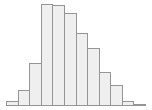1427 (100%) 0 (0%)
2 PESOKG [numeric] Mean (sd) : 45 (10.7) min < med < max: 21 < 43 < 100 IQR (CV) : 14 (0.2) 65 distinct values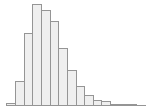1427 (100%) 0 (0%)
3 TORACECM [numeric] Mean (sd) : 75.6 (7.8) min < med < max: 57 < 74 < 104 IQR (CV) : 10 (0.1) 44 distinct values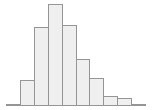1427 (100%) 0 (0%)
4 CRANIOCM [numeric] Mean (sd) : 54.8 (1.6) min < med < max: 50 < 55 < 60 IQR (CV) : 2 (0) 11 distinct values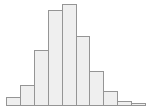1427 (100%) 0 (0%)
5 BISACROM [numeric] Mean (sd) : 34.5 (3) min < med < max: 23 < 34 < 46 IQR (CV) : 4 (0.1) 21 distinct values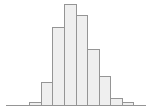1427 (100%) 0 (0%)
6 BITROCAN [numeric] Mean (sd) : 26.3 (2.8) min < med < max: 20 < 26 < 38 IQR (CV) : 4 (0.1) 18 distinct values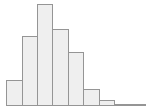1427 (100%) 0 (0%)
7 SPANCM [numeric] Mean (sd) : 153.6 (11.2) min < med < max: 123 < 153 < 184 IQR (CV) : 16 (0.1) 60 distinct values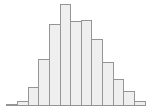1427 (100%) 0 (0%)

Generated by summarytools 0.9.6 (R version 4.0.2)
2020-09-10

MLA.explor.pairs(X,pch=".")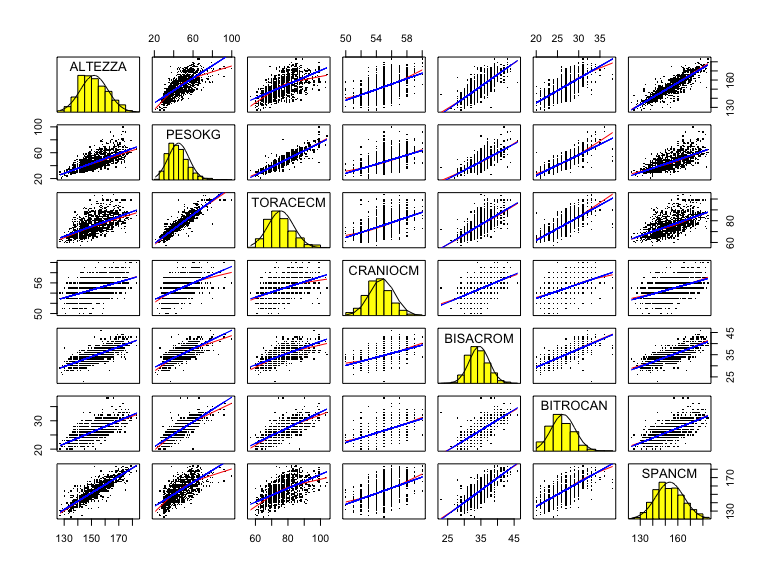"First multivariate moment"
 "First multivariate moment"
print(round(colMeans(X),2))
 ALTEZZA   PESOKG TORACECM CRANIOCM BISACROM BITROCAN   SPANCM
151.95    44.98    75.63    54.76    34.52    26.34   153.60 
"Second multivariate centered moment"
 "Second multivariate centered moment"
print(round(cov(X),2))
         ALTEZZA PESOKG TORACECM CRANIOCM BISACROM BITROCAN SPANCM
ALTEZZA   101.90  78.37    46.30     7.82    22.62    20.80 100.75
PESOKG     78.37 114.99    76.35     9.32    23.97    25.00  82.67
TORACECM   46.30  76.35    61.00     6.12    16.11    16.74  50.48
CRANIOCM    7.82   9.32     6.12     2.64     2.41     2.20   8.59
BISACROM   22.62  23.97    16.11     2.41     8.84     6.33  25.88
BITROCAN   20.80  25.00    16.74     2.20     6.33     7.76  22.08
SPANCM    100.75  82.67    50.48     8.59    25.88    22.08 125.18

The last matrix is the sample covariance matrix, so that e.g. cov(X)[2,2]=114.99 is the sample variance of second variable, while cov(X)[2,3]=76.35 is the covariance between second and third variable. For regression:

round(cov(X)[2,3]/cov(X)[3,3],2)
 1.25

is the regression coefficient of the second variable as a function of the third.

round(cov(X)[2,3]/cov(X)[3,3],2)
 1.25

### 1.5.2 Information of correlation matrix

R=cor(X)
round(R,2)
         ALTEZZA PESOKG TORACECM CRANIOCM BISACROM BITROCAN SPANCM
ALTEZZA     1.00   0.72     0.59     0.48     0.75     0.74   0.89
PESOKG      0.72   1.00     0.91     0.54     0.75     0.84   0.69
TORACECM    0.59   0.91     1.00     0.48     0.69     0.77   0.58
CRANIOCM    0.48   0.54     0.48     1.00     0.50     0.49   0.47
BISACROM    0.75   0.75     0.69     0.50     1.00     0.76   0.78
BITROCAN    0.74   0.84     0.77     0.49     0.76     1.00   0.71
SPANCM      0.89   0.69     0.58     0.47     0.78     0.71   1.00

R[2,3]=0.91 is the linear correlation between second and third variable.

### 1.5.3 Information of inverse of correlation matrix

Multiple and partial regression

C=solve(cor(X))
round(C,2)
         ALTEZZA PESOKG TORACECM CRANIOCM BISACROM BITROCAN SPANCM
ALTEZZA     5.97  -1.95     1.41    -0.08    -0.24    -0.82  -3.99
PESOKG     -1.95   9.69    -6.13    -0.46    -0.11    -1.74   0.14
TORACECM    1.41  -6.13     6.58    -0.02    -0.67    -0.48   0.03
CRANIOCM   -0.08  -0.46    -0.02     1.46    -0.21     0.00  -0.12
BISACROM   -0.24  -0.11    -0.67    -0.21     3.58    -0.85  -1.40
BITROCAN   -0.82  -1.74    -0.48     0.00    -0.85     4.12  -0.04
SPANCM     -3.99   0.14     0.03    -0.12    -1.40    -0.04   5.62

Since C[2,2]=9.69, then $$R^2_{2.134567}=1-\frac{1}{c_{22}}=0.897$$

( Multiple $$R^2$$ index of the second variable with respect to remainings ones).

And since C[2,3]=-6.13, then

$$r_{23.14567}=\frac{-c_{23}}{\sqrt{c_{22} c_{33}}}=0.767$$

is the partial correlation between second and third variable, keeping constants all the other ones.

I skip here formulas for two first moments of multivariate observations